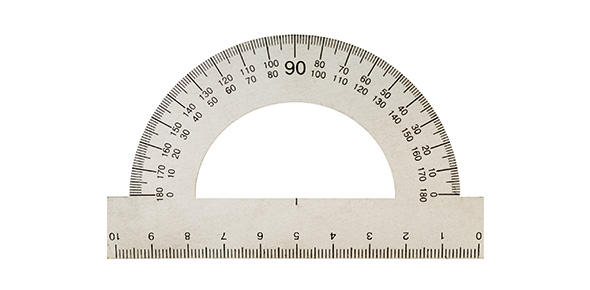# Measurement

8 Questions | Total Attempts: 63SettingsBenchmark(s): A, B, C * Select appropriate units for perimeter, area, weight, volume (capacity), time and temperature * Know that the number of units is inversely related to the size of the unit for any item being measured * Develop common references for units of measure for length, weight, volume (capacity), and time to make comparisons and estimates

• 1.
Which unit of measurement is associated with area?
• A.

A.m. / p.m.

• B.

Regular units

• C.

Square units

• D.

C* / F*

• E.

Cubic units

• 2.
Which unit of measurement is associated with volume?
• A.

A.m. / p.m.

• B.

Regular units

• C.

Square units

• D.

C* / F*

• E.

Cubic units

• 3.
Which unit of measurement is associated with perimeter?
• A.

A.m. / p.m.

• B.

Regular units

• C.

Square units

• D.

C* / F*

• E.

Cubic units

• 4.
Which unit of measurement is associated with time?
• A.

A.m. / p.m.

• B.

Regualr units

• C.

Square units

• D.

C* / F*

• E.

Cubic units

• 5.
Which unit of measurement is associated with temperature?
• A.

A.m. / p.m.

• B.

Regualr units

• C.

Square units

• D.

C* / F*

• E.

Cubic units

• 6.
If I wanted to fill an aquarium with water, which sized container would be best to use?
• A.

Ounce

• B.

Cup

• C.

Pint

• D.

Quart

• E.

Gallon

• 7.
Which item weighs about 1 gram?
• A.

Paperclip

• B.

Pencil

• C.

Book

• D.

Computer

• E.

Car

• 8.
I'm filling up my kids' sandbox with sand. I'm using a 1 cup container. If I switch to a 1 gallon container, my number of scoops will be __________.
• A.

Less

• B.

More

• C.

Same

• D.

All of the above

• E.

Not enough information

Related Topics